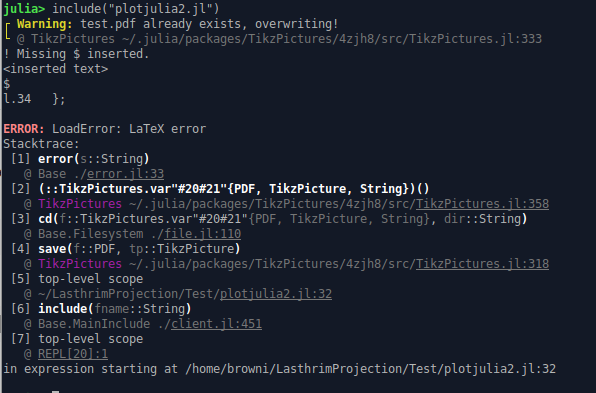# Probability Outcomes Plot with JULIA for Fair Coin Tossing

Hi all,

I want to plot the possible outcomes of tossing a fair coin for n times. If three times tossed then it should be like this graph: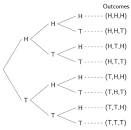Which package in Julia can make this kind of graph?

You could use GraphMakie.jl in the Makie or GraphRecipes.jl in the Plots ecosystem.

However I think using Julia plotting libraries makes the most sense when you’re plotting data. Your example looks like you want to explain a concept with a curated graphic, for that I’d recommend to go for a tool like tikz or inkscape rather than a plotting lib.

1 Like

I want to use Julia so it can calculate the probability and the outcome sets too.

Tikz is possible to graph it, but how about the numerical part? if I want to toss a coin 10 times. Julia should be able to calculate it fast with another package right?

Yes, if you want to visualize a specific sample it makes sense to use a plotting lib. I think you can get fine results using both mentioned packages.
In your original post the graphic includes all possible outcomes for 3 throws, without any “dynamic” data, which is why I suggested a more static approach.

1 Like

To get the probabilities, you can use the analytical expression:

P_k(n) = \choose{k}{n} \cdot \left(\frac{1}{2}\right)^n


or

probability(k, n) = nchoosek(n, k)*(1//2)^n

1 Like

I can’t find the perfect sentences so people could understand what I want. If it is finish I will post it here.

I suppose that you intend to vizualize the binary tree, associated to the experiment consisting in n independent Bernoulli trials. A Bernoulli trial has two mutual exclusive outcomes: success and failure. The probability p of the succes is the same any time when you repeat the trial. In your case p=1/2. In an experiment with n independent trials one is interested in the number if successes (or failures). The random variabile X that records the number k of successes in n trials has the Binomial distribution, and P(X=k)={n \choose k} p^k (1-p)^{n-k}. In your case is given by the formula given by @gustaphe.

1 Like

I have tried with an ugly graph like this: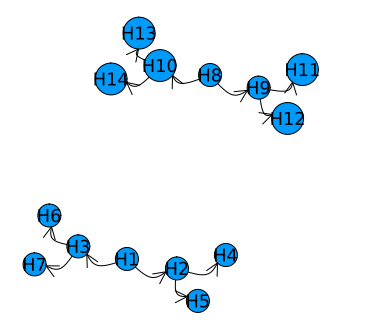using GraphRecipes, Plots
gr()
default(size=(800, 400))

g = [0  1  1  0  0  0  0  0  0  0  0  0  0  0;
0  0  0  1  1  0  0  0  0  0  0  0  0  0;
0  0  0  0  0  1  1  0  0  0  0  0  0  0;
0  0  0  0  0  0  0  0  0  0  0  0  0  0;
0  0  0  0  0  0  0  0  0  0  0  0  0  0;
0  0  0  0  0  0  0  0  0  0  0  0  0  0;
0  0  0  0  0  0  0  0  0  0  0  0  0  0;
0  0  0  0  0  0  0  0  1  1  0  0  0  0;
0  0  0  0  0  0  0  0  0  0  1  1  0  0;
0  0  0  0  0  0  0  0  0  0  0  0  1  1;
0  0  0  0  0  0  0  0  0  0  0  0  0  0;
0  0  0  0  0  0  0  0  0  0  0  0  0  0;
0  0  0  0  0  0  0  0  0  0  0  0  0  0;
0  0  0  0  0  0  0  0  0  0  0  0  0  0;]
graphplot(g, fontsize=12, names="H".*string.(1:14), nodeshape=:circle)



The problems:

1. I cannot name each node as H or T, with H,T won’t work
2. I really want to create like the image I post at the first post… there are seems to many Graphs packages in Julia, LightGraphs, GraphRecipes, … maybe they are more suitable to Optimization, Network Flows branches.

I might be able to use Tikz to draw the wanted image, but I think Julia should be able to do it too.

One possibility is to use Julia to generate the TikZ code:

using TikzPictures

function branch(result, n, i=1, prev=[])
sofar = [prev; result]
indent = "  "^i
distance = 5 * 2^(n-i)
if i == n
fullbranch = join(sofar, ",")
prob = "\$p = \\frac{1}{$(2^n)}\$" return """$(indent)child [sibling distance=$(distance)ex]$(indent)  { node [anchor=west] { $result \$\\cdots\$($fullbranch)\\; $prob } }""" else return """$(indent)child [sibling distance=$(distance)ex] { node {$result}
$(branch(:T, n, i+1, sofar))$(branch(:H, n, i+1, sofar))
$(indent)}""" end end function tree(n) return """ \\node {}$(branch(:T, n))
$(branch(:H, n));""" end TikzPicture(options="grow=right", tree(3))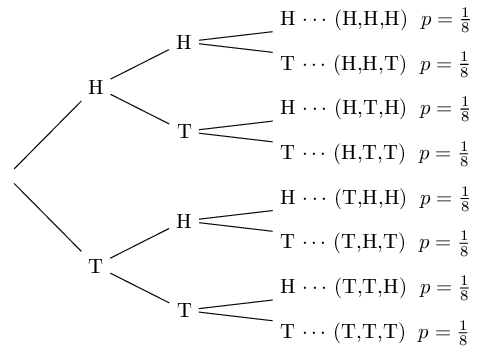6 Likes To define a binary tree of depth h=3, the adjacency matrix must have the size (15,15). Your matrix is (14, 14). This function creates the ajacency matrix of a perfect binary tree of depth h: function get_adjacency(h::Int) nn = 2^(h+1)-1 #number of nodes A = zeros(Int64, nn, nn); j=2 for i = 1:2^h-1 A[i, j] = 1 A[i, j+1] =1 j=j+2 end return A end  To assign a position to each tree node, install NetworkLayout.jl. Then with: using Graphs, NetworkLayout A = get_adjacency(3) G = DiGraph(A); node_pos = buchheim(G.fadjlist)  you get a binary tree placed vertically. By default, the root is at the point (0,0). To get an horizontally displayed tree, rotate the points in the vector node_pos around the root with an angle of π/2. The rotation matrix is [0 -1; 1;0]. Having the new node position you can now plot the corresponding binary tree. 2 Likes I didn’t know the logic behind matrix of size (15,15) for h=3, thanks @empet . I just go with my own thinking for matrix 14x14. Julia is amazing Wow @sijo this is what I am looking for. Thanks a bunch! 1 Like I get this when run the code at REPL: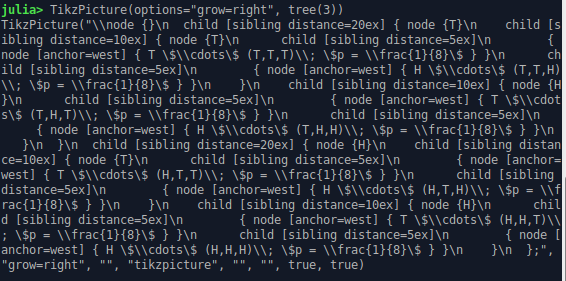why it won’t show the image? The REPL cannot show images… You should see the image if you run the code in a Jupyter notebook. Or if you save the result in a variable tp, you can call save(PDF("filename"), tp) to output a PDF file (see the TikzPictures.jl documentation for more details), (To put the image here on Discourse I used the Linux convert tool to convert the PDF to PNG.) The adjacency matrix of a graph has the size (nn, nn), where nn is the number of nodes. Now count the nodes in the above plotted binary tree and you get 15. I think I have to put L """ in the right place, the pdf generated but it is empty: using TikzPictures function branch(result, n, i=1, prev=[]) sofar = [prev; result] indent = " "^i distance = 5 * 2^(n-i) if i == n fullbranch = join(sofar, ",") prob = "\$p = \\frac{1}{$(2^n)}\$"
return  """
$(indent)child [sibling distance=$(distance)ex]
$(indent) { node [anchor=west] {$result \$\\cdots\$ ($fullbranch)\\;$prob } }"""
else
return  """
$(indent)child [sibling distance=$(distance)ex] { node {$result}$(branch(:T, n, i+1, sofar))
$(branch(:H, n, i+1, sofar))$(indent)}"""
end
end

function tree(n)
return """
\\node {}
$(branch(:T, n))$(branch(:H, n));"""
end

tp = TikzPicture(L""" options="grow=right", tree(3)""")

save(PDF("test"), tp)


No you shouldn’t add any L: all the dollars and backslashes in my code are already escaped. So replace

tp = TikzPicture(L""" options="grow=right", tree(3)""")


with

tp = TikzPicture(options="grow=right", tree(3))


and it should work.

Following the steps I outlined above, I defined the binary tree associated
to a Bernoulli experiment consisting in 4 trials. 1 encodes the trial success, while 0, the failure.
The outcomes of this experiment are 4-length bitstrings returned by this function:

function bit_strings(h::Int)
#returns the vector of h-length bitstrings
vbstr = String[]
for i = 0:2^h-1
s = bitstring(Int8(i)) #Int8 is sufficient for h=4, 5, 6, 7
push!(vbstr, s[length(s)-h+1:end])
end
return vbstr
end


3 Likes

I tried with Jupyter Notebook too why this code can’t work ? Neither REPL nor notebook works.

My Julia: version 1.7.3
status:

Status ~/LasthrimProjection/Test/Project.toml
[bd48cda9] GraphRecipes v0.5.12
[86223c79] Graphs v1.8.0
 ImplicitEquations v1.0.9
[b964fa9f] LaTeXStrings v1.3.0
[f0f68f2c] PlotlyJS v0.18.10
[91a5bcdd] Plots v1.38.0
[438e738f] PyCall v1.94.1
[d330b81b] PyPlot v2.11.0